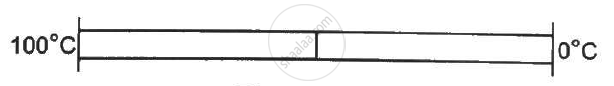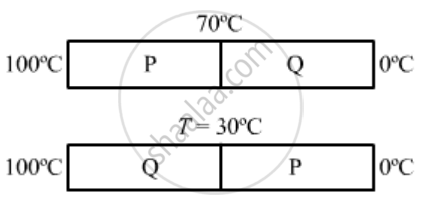Advertisement Remove all ads

# The Two Rods Shown in Figure (28-e6) Have Identical Geometrical Dimensions. They Are in Contact with Two Heat Baths at Temperatures 100°C and 0°C. - Physics

Sum

The two rods shown in following figure  have identical geometrical dimensions. They are in contact with two heat baths at temperatures 100°C and 0°C. The temperature of the junction is 70°C. Find the temperature of the junction if the rods are interchanged.Advertisement Remove all ads

#### SolutionAs the rods are connected in series, the rate of flow of heat will be same in both the cases.

ase 1:
Rate of flow of heat is given by  {dQ}/dt  = (KA DeltaT)/l

Rate of heat flow in rod P will be same as that in rod Q.

∴ {K_pxxAxx(100 - 70)}/l ="(KQxx Axx(70 - 0))/l

⇒ 30 Kp = 70K_Q

⇒ K_Q = 3/7 K_p ......................(1)

Case 2:
Again, the rate of flow of heat will be same in rod P and Q.

∴ (K_QxxAxx(1100-T))/l  = (K_pxxAxx(T - 0))/(l)

100 K_Q - K_QT = K_pT

100K_Q - K_QT = 70/30K_QT  .........{using (i)}

100- T = 7/3T

100 = 10/3 t

⇒ T = 30^circ C

Concept: Thermal Expansion of Solids
Is there an error in this question or solution?
Advertisement Remove all ads

#### APPEARS IN

HC Verma Class 11, Class 12 Concepts of Physics Vol. 2
Chapter 6 Heat Transfer
Q 29 | Page 100
Advertisement Remove all ads
Advertisement Remove all ads
Share
Notifications

View all notifications

Forgot password?